# Boolean algebra facts for kids

Kids Encyclopedia Facts
(Redirected from Boolean logic)

Boolean algebra is algebra for binary (0 means false and 1 means true). It uses normal maths symbols, but it does not work in the same way. It is named after its creator George Boole.

## NOT gate

NOT
0 1
1 0

The NOT operator is written with a bar over numbers or letters like this: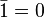$\bar{1} = 0$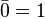$\bar{0} = 1$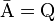$\bar{\mbox{A}} = \mbox{Q}$

It means the output is not the input.

## AND gate

AND 0 1
0 0 0
1 0 1

The AND operator is written as$\cdot$ like this: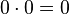$0 \cdot 0 = 0$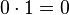$0 \cdot 1 = 0$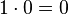$1 \cdot 0 = 0$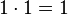$1 \cdot 1 = 1$

The output is true only if one and the other input is true.

## OR gate

OR 0 1
0 0 1
1 1 1

The OR operator is written as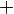$+$ like this: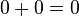$0 + 0 = 0$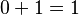$0 + 1 = 1$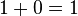$1 + 0 = 1$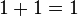$1 + 1 = 1$

One or the other input can be true for the output to be true.

## XOR gate

XOR 0 1
0 0 1
1 1 0

XOR basically means "exclusive or", meaning one input or the other must be true, but not both.

The XOR operator is written as$-$ like this: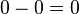$0 - 0 = 0$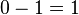$0 - 1 = 1$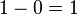$1 - 0 = 1$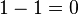$1 - 1 = 0$

To make it more simple, one or the other input must be true, but not both.

## Identities

Different gates can be put together in different orders: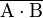$\overline{\mbox{A} \cdot \mbox{B}}$ is the same as an AND then a NOT. This is called a NAND gate.

It is not the same as a NOT then an AND like this: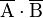$\overline{\mbox{A}} \cdot \overline{\mbox{B}}$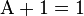$\mbox{A} + 1 = 1$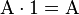$\mbox{A} \cdot 1 = \mbox{A}$

which is called XOR identity table

XOR 1 0 Any
1 TRUE 0 0
0 0 0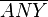$\overline{ANY}$
Any 0$\overline{ANY}$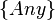$\{Any\}$

, if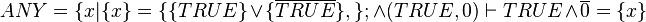$ANY=\{x|\{x\}=\{\{TRUE\}\or\{\overline{TRUE}\}, \};\and (TRUE, 0) \vdash TRUE \and \overline{0} = \{x\}$.

or if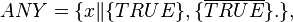$ANY=\{x \|\{TRUE\}, \{\overline{TRUE}\} .\},$=TRUE, TRUE.,

## DeMorgan's laws

Augustus De Morgan found out that it is possible to change a$+$ sign to a$\cdot$ sign and make or break a bar. See the 2 examples below: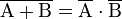$\overline{\mbox{A} + \mbox{B}} = \overline{\mbox {A}} \cdot \overline{\mbox{B}}$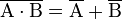$\overline{\mbox{A} \cdot \mbox{B}} = \overline{\mbox {A}} + \overline{\mbox{B}}$

"Make/break the bar and change the sign."

## Images for kidsBoolean algebra Facts for Kids. Kiddle Encyclopedia.